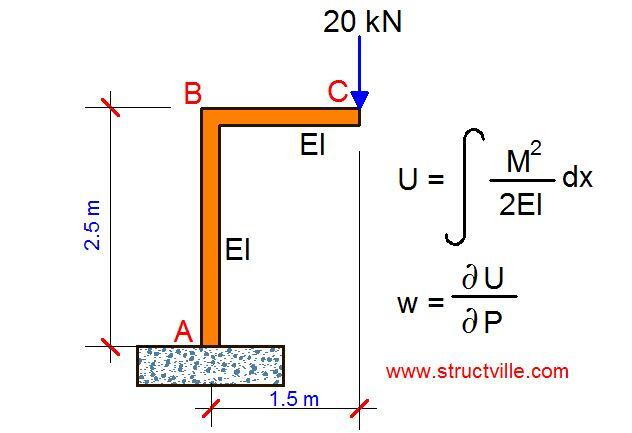# Deflection of Elastic Systems Using Castigliano’s TheoremCastigliano’s method for calculating deflections is an application of his second theorem, which states that if the strain energy of a linearly elastic structure can be expressed as a function of generalised force Pi then the partial derivative of the strain energy with respect to generalised force gives the generalised displacement wi in the direction of Pi.

The second theorem of Castigliano is applicable to linearly elastic (Hookean material) structures with constant temperature and unyielding supports.

In general, this is given by;

wi = ∂U/∂Pi

The strain energy stored in a linear elastic system due to bending is given by;

Solved Example
For the frame loaded as shown below, let us find the vertical deflection at point C due to bending using Castigliano’s theorem.

Solution
Section BC
Mx = -20x

U1 = ∫[(-20x)2/2EI] dx  =  ∫ -400x2/2EI = -400x3/6EI
Knowing that the limit x = 1.5m;
U1 = 225/EI

Section AB
My = (-20 × 1.5m) = 30 kNm

U2 = ∫[(-30)2/2EI] dy  =  ∫ -900/2EI = -900y/2EI
Knowing that the limit y = 2.5m;
U2 = 1125/EI

Total strain energy = U1 + U2 = (225/EI) + (1125/EI) = 1350/EI

Let the vertical deflection at point C be δv

Work done by the externally applied load = 1/2(P × δ)
Work done = Strain energy stored in the system

1/2(20 × δ) = 1350/EI

δv = 135/EI metres